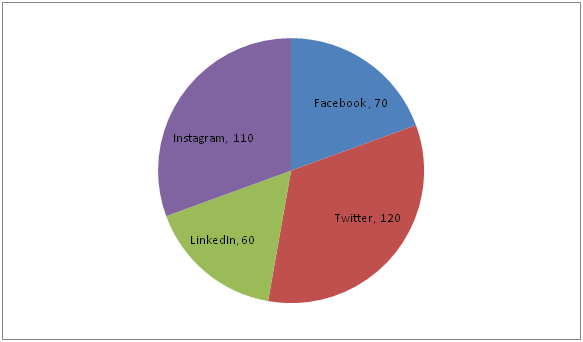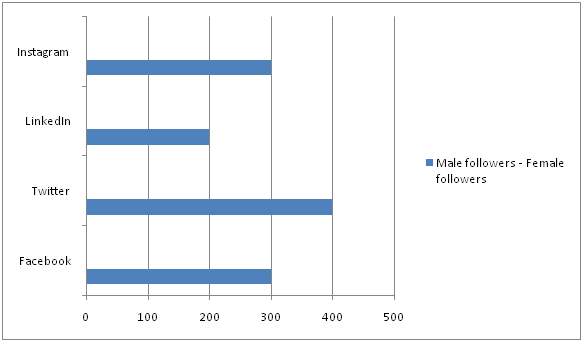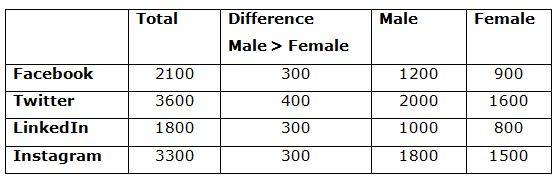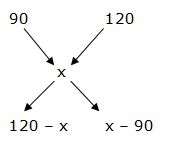# LIC HFL Assistant Manager Quantitative Aptitude Questions – (Day-07)

Dear Aspirants, Our IBPS Guide team is providing new series of Quantitative Aptitude Questions for LIC HFL Assistant Manager 2019 so the aspirants can practice it on a daily basis. These questions are framed by our skilled experts after understanding your needs thoroughly. Aspirants can practice these new series questions daily to familiarize with the exact exam pattern and make your preparation effective.

Check here for LIC HFL Mock Test 2019

Check here for IBPS RRB PO Mains Mock Test 2019

Check here for IBPS RRB Clerk Mains Mock Test 2019

### Click Here to Subscribe Crack High Level Puzzles & Seating Arrangement Questions PDF 2019 Plan

[WpProQuiz 7152]

Directions (1 – 5): Study the following information carefully and answer the questions given below.

Pie- chart given below shows the degree distribution of followers of Priyanka from different social media platforms. Bar chart given below shows the difference between male and female followers. In every social media platform, male followers of Priyanka are more than her female followers.

Note: Total followers are 10800 and all the followers are different persons.1) Find the ratio of male followers of Priyanka on Facebook to the female followers on Twitter?

a) 2: 3

b) 3: 4

c) 4: 5

d) 5: 6

e) 6: 7

2) If 70% of the male followers and 80% of the female followers of Priyanka in LinkedIn are from India then the males followers who are not form India is approximately what percent of females followers who are from India?

a) 43%

b) 45%

c) 47%

d) 51%

e) 49%

3) If the ratio of the total number of photos uploaded by Priyanka on Instagram and Facebook to the total number of her followers on Facebook and Instagram is 1: 9. Find the total number of photos uploaded by Priyanka on Facebook and Instagram.

a) 300

b) 400

c) 500

d) 600

e) 900

4) Find the difference in number of male followers of Priyanka on Instagram to the number of her female followers on Twitter.

a) 100

b) 200

c) 300

d) 400

e) 500

5) The average age (in years) of Priyanka’s followers on Facebook, Twitter, LinkedIn and Instagram is 16, 24, 28 and 12 respectively. Find the approximate average age of Priyanka’s followers on social media? (Consider the given four platforms)

a) 19.5 years

b) 20.5 years

c) 18.5 years

d) 21.5 years

e) 22.5 years

6) 40 % of goods are sold at 10 % loss while the remaining is sold at 20 % profit. If there is total profit of Rs. 640, then the worth of goods sold is?

a) Rs. 8000

b) Rs. 9500

c) Rs. 8600

d) Rs. 9200

e) None of these

7) 15 men or 45 women can do a piece of work in 24 days. In how many days will 32 men and 48 women can do double of work?

a) 15 days

b) 12 days

c) 18 days

d) 10 days

e) None of these

8) P, Q and R started a business by investing in the ratio of 4: 7: 10. After 8 months, P invested 25 % more than the initial investment but R withdrew Rs. 10000. Find the initial investment of R, if the profit share of P, Q and R is in the ratio of 13: 21: 28.

a) Rs. 42000

b) Rs. 56000

c) Rs. 50000

d) Rs. 64000

e) None of these

9) If the compound interest on certain sum for 3 years at 12 % per annum is Rs. 24295.68, then find the corresponding simple interest.

a) Rs. 22500

b) Rs. 21600

c) Rs. 23700

d) Rs. 24900

e) None of these

10) A bag contains (x + 2) blue, 5 red and 7 yellow colour balls. If two balls are taken random and the probability of getting both are yellow colour balls is 1/10, then find the difference between the number of blue colour balls and red colour balls.

a) 3 balls

b) 4 balls

c) 1 ball

d) 2 balls

e) None of these

Directions (1 – 5):Required ratio = 1200: 1600

= 3: 4

Male followers who are not from India = {(100 – 70)/100} * 1000

= (30/100) * 1000 = 300

Female followers who are from India = (80/100) * 800 = (4/5) * 800 = 640

Required percentage = (300/640) * 100

= (30/64) * 100 = (3000/64) = (750/16) = 47% (approx)

Total followers of Priyanka on Facebook and Instagram = 5400

Let the number of photos uploaded = x

The number of photos uploaded: Total number of followers = 1: 9

x: 5400 = 1: 9

x/5400 = 1/9

x = 600

Required difference = (1800 – 1600) = 200

Total age of followers = Number of followers * Average age of followers

Total age of followers = 2100 * 16

Total age of followers = 33600

Total age of followers = Number of followers * Average age of followers

Total age of followers = 3600 * 24

Total age of followers = 86400

Total age of followers = Number of followers * Average age of followers

Total age of followers = 1800 * 28

Total age of followers = 50400

Instagram:

Total age of followers = Number of followers * Average age of followers

Total age of followers = 3300 * 12

Total age of followers = 39600

Required average = (Total age of followers on all four platform)/ (total followers on all four platform)

Required average = (33600 + 86400 + 50400 + 39600)/ (10800)

Required average = 19.5 years (approx)

Directions (6-10) :Given,

= > (120 – x)/(x – 90) = (2/3)

= > 360 – 3x = 2x – 180

= > 540 = 5x

= > x = 540/5 = 108

Overall profit % = 8 %

(8/100) * Cost price = 640

Cost price = (640 * 100)/8 = Rs. 8000

15 men = 45 women

1 men = 3 women

32 m + 48 w = 32 m + 16 m = 48 m

Men            days

15               24

48               ?

(15 * 24) = (48 * x)

x = 15/2

Double the work in = (15/2) * 2 = 15 days

The share of P, Q and R,

[4x * 8 + (4x * (125/100) * 4]: [7x * 12]: [10x * 8 + (10x – 10000) * 4] = 13: 21: 28

= > [32x + 20x]: [84x]: [80x + 40x – 40000] = 13: 21: 28

= > [52x]: [84x]: [120x – 40000] = 13: 21: 28

According to the question,

52x/(120x – 40000) = (13/28)

120x – 40000 = 112x

8x = 40000

x = 5000

The initial investment of R = 10x = Rs. 50000

Let the sum of money be x,

x * [(1 + (12/100))3 – 1] = 24295.68

x * [(112/100)3 – 1] = 24295.68

x * [(21952/15625) – 1] = 24295.68

x * (6327/15625) = 24295.68

x = 24295.68 * (15625/6327) = Rs. 60000

Corresponding SI:

SI = (60000 * 3 * 12)/100 = Rs. 21600

7C2/ (x+14)C2 = 1/10

[(7 * 6)/(1 * 2)]/[(x + 14) (x + 13)/(1 * 2)] = 1/10

(42 * 10) = x2 + 13x + 14x + 182

420 = x2 + 27x + 182

x2 + 27x – 238 = 0

(x – 7) (x + 34) = 0

x = 7, -34 (negative value will be eliminated)

Required difference = 9 – 5 = 4 balls